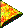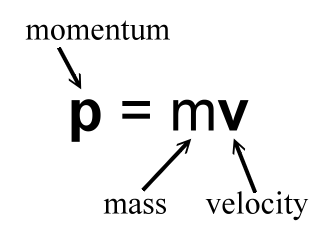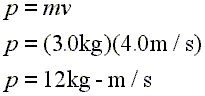# Introductory Momentum Equations, DefinitionBasic definition:

Momentum is equal to mass times velocity. It is symbolized with a small p. Mass is symbolized with a small m, velocity with a small v.

 Momentum equals mass times velocity.Here is an example calculation. Notice that the unit for momentum is kg-m/s.Line 1: Definition of momentum. Line 2: Enter example values for mass and velocity. Line 3: Calculate the resultant momentum.

So an object with a mass of 3.0 kg that is traveling at a velocity of 4.0 m/s has a momentum of 12 kg-m/s.

Momentum is a vector. The size of this vector is equal to the value of the mass times the velocity. This size of the momentum is 12 kg-m/s in the above example. The direction of the momentum vector is the same as the direction of the velocity.

We can use algebra to rearrange the momentum equation. First we have:

p = mv (Momentum equals mass times velocity.)

Which rearranges to these equations:

m = p/v (Mass equals momentum divided by velocity.)

v = p/m (Velocity equals momentum divided by mass.)

Here are some small EZ Spread Sheets that calculate with the above momentum formulas:

p = mv

m = p/v

v = p/m

Check your understanding by trying some of these problems:

Related Problems
   

Custom Search